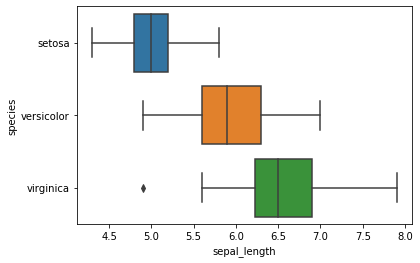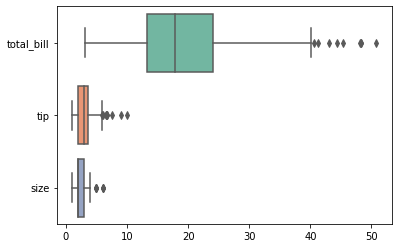Open in App
Not now

# Horizontal Boxplots with Seaborn in Python

• Difficulty Level : Hard
• Last Updated : 12 Nov, 2020

Prerequisite: seaborn

The Boxplots are used to visualize the distribution of data which is useful when a comparison of data is required. Sometimes, Boxplot is also known as a box-and-whisker plot. The box shows the quartiles of dataset and whiskers extend to show rest of the distribution. In this article, we are going to implement the Horizontal boxplot with seaborn using python.

## Horizontal Box plots

Seaborn uses the boxplot() method to draw a boxplot. We can turn the boxplot into a horizontal boxplot by two methods first, we need to switch x and y attributes and pass it to the boxplot( ) method, and the other is to use the orient=”h” option and pass it to the boxplot() method.

Method 1: Switching x and y attribute

## Python3

 `# import library & dataset ` `import` `seaborn as sns ` ` `  ` `  `df ``=` `sns.load_dataset(``'iris'``) ` ` `  `# Just switch x and y ` `sns.boxplot(y``=``df[``"species"``], x``=``df[``"sepal_length"``]) `

Output:Horizontal Boxplot

Method 2: Using orient = h

## Python3

 `# import library & dataset ` `import` `seaborn as sns ` ` `  ` `  `tips ``=` `sns.load_dataset(``"tips"``) ` `ax ``=` `sns.boxplot(data``=``tips, orient``=``"h"``, palette``=``"Set2"``) `

Output :Horizontal Boxplot

My Personal Notes arrow_drop_up
Related Articles